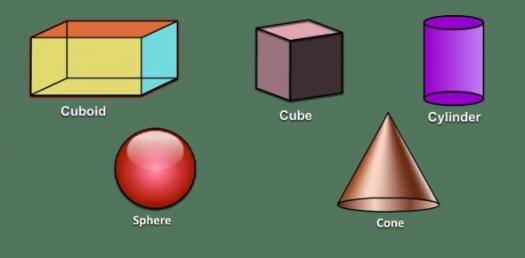# Can You Identify 2D And 3D Shapes? Trivia Questions Quiz

5 Questions | Attempts: 2402
ShareSettingsCan You Identify 2d And 3D Shapes? This trivia quiz is perfect for helping people learn about different shapes and how to measure them. If you think that you know enough to tackle it, all you have to do is give it a try. All the best as you tackle and check out other quizzes just like it!

• 1.
A Circle and Pentagon are both considered POLYGONS
• A.

True

• B.

False

• 2.
WHAT AM I? I have 4 corners and 2 sets of sides that are equal in size.   I have two sets of parallel sides. I have a set of acute and obtuse angles.   WHAT AM I ??? What am I?
• A.

TRAPEZOID

• B.

RHOMBUS

• C.

RECTANGLE

• D.

PARALLELOGRAM

• 3.
Of the choices below, which shapes are considered two dimensional?
• A.

Circle

• B.

Trapezoidal Prism

• C.

Edge

• D.

Sphere

• E.

Rhombus

• F.

Cube

• G.

Hexagon

• H.

Paralleogram

• I.

Cone

• J.

Triangular Prism

• 4.
USING MATHEMATICAL LANGUAGE AND TERMINOLOGY, Explain a difference between A SEPTAGON and A DODECAGON? Refer to the number of sides or angles within each shape.
• 5.
Of the choices below, which shapes are considered Three dimensional?
• A.

Cylinder

• B.

Trapezoid

• C.

Decagon

• D.

Rectangular Prism

• E.

Square

• F.

Cube

• G.

Isosceles Triangle

• H.

Pentagonal Prism

• I.

Cone

## Related TopicsBack to top
×

Wait!
Here's an interesting quiz for you.